# High School Math : Simplifying and Expanding Quadratic Equations

## Example Questions

### Example Question #1 : Quadratic Equations And Inequalities

Solve the following equation using the quadratic form: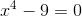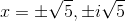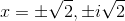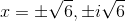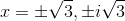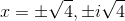Explanation:

Factor the equation and solve: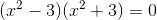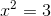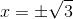or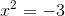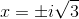### Example Question #2 : Quadratic Equations And Inequalities

Solve the following equation using the quadratic form: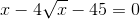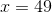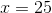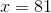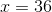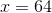Explanation:

Factor the equation and solve: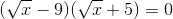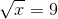or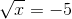This has no solutions.

Therefore there is only one answer: